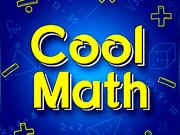Cool Math Game# Cool Math Game Online - Play Fun Kids Brain Puzzle Games

This arithmetic and logic game will drive you and your enthusiastic friends to excel in mathematics. Cool Math is the newest hit from the world of fun online games. This free online game is simple to play but difficult to master. The algorithm in this game allows the users to play and train his brain with ongoing levels that are getting heavier and heavier as you go further. Every level contains ten random exercises (difficulty depending on the level) witch you need to answer correctly if you want to go further in the highest level. Have fun!

11,273 play times

## How to Play Cool Math Game

Touch your devices or use the mouse if available.

## Mathematics is a Science through Logical Definitions

Mathematics itself is the more or less beloved and popular science that has arisen from the study of figures (triangles, squares, rectangles, ...) as well as from doing calculations with numbers. For mathematics, there is no generally accepted definition that is accepted by everyone today. Today, mathematics is usually outlined and described as a science, which examines itself through its own logical definitions and thereby created abstract structures using logic to look into its properties as well as patterns. Mathematics is the basis of nearly all modern sciences. Using mathematics, theories are tested and falsified. Hardly any student likes math, because math is extremely boring and relatively sluggish. Mathematical definitions, equations and curve discussions are the horror of many students. Here at KidsAndOnlineGames.com, you can now refresh your math knowledge online and simply learn math by playing these games. Learning how to add and subtract with these great mathematics online games is a lot of fun!

## Calculate the size of each side of the triangle

Another example from geometry is the right triangle, which was also known in ancient Greece. It was Pytagoras who could first calculate the size of each side of the triangle when only two sites were known. For this, you have to know the elementary basics of arithmetic but also the extraction of roots. In memory of the Greek mathematician and philosopher Pytagoras, the formula is named the Pytagoras theorem. For calculations, the sum of the squares of the two shorter sides equals the square of the hypotenuse.

Tags: CoolMathMathematics